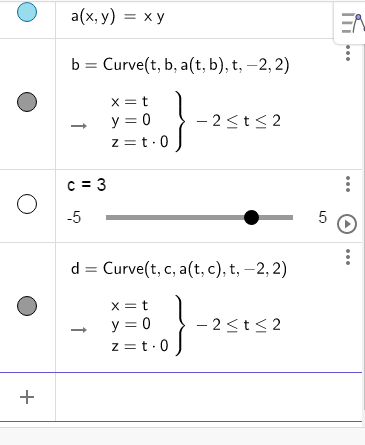# Slider variable value not adjusted correctly

Derrick Wigglesworth shared this problem 7 months ago
New

I have only seen this issue happen with the 3d graphing tool. I don't know if it exists in the 2d environment as well.

A summary of the issue: Sometime when I create a slider for a variable, say b, and then later use that variable in a formula, equation, or function, rather than use the value of b assigned by the slider, the applet just treats it as 0.

Here is a way to consistently recreate the issue:

1. Define a function of two variables, say f(x,y)=xy.
2. Make a slider, say by typing b=2.
3. Now attempt to make a curve on the surface that goes in the x direction, at a y=value of b: Curve(t,b,f(t,b),t,-10,10)
4. When you hit enter, the curve will use b=0, rather than b=2.1

Perhaps it is a good idea to make the slider first, before defining the function or change the order in construction protocol?1

I think there is a little bug

try

f(x,y)=x y

curve(t,b,f(t,b),t,0,4) (the curve is created being b equal to 0; wrong)

b=3 (the curve is redefined being b=3)1

For me it creates it with b=1 (OK)1the name b for curve is automatic; then error in line 2 at opening

curve d is right after save and open with ver5

Files: foro.ggb1

It definitely seems like a bug. I did manage to get things working correctly following hawe's suggestion and making the slider last.